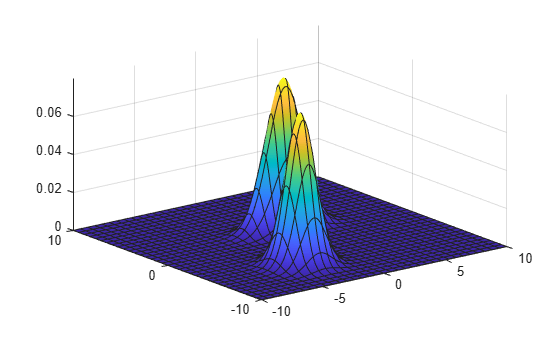Documentation

# pdf

Probability density function for Gaussian mixture distribution

## Syntax

``y = pdf(gm,X)``

## Description

example

````y = pdf(gm,X)` returns the probability density function (pdf) of the Gaussian mixture distribution `gm`, evaluated at the values in `X`.```

## Examples

collapse all

Create a `gmdistribution` object and compute its pdf values.

Define the distribution parameters (means and covariances) of a two-component bivariate Gaussian mixture distribution.

```mu = [1 2;-3 -5]; sigma = [1 1]; % shared diagonal covariance matrix```

Create a `gmdistribution` object by using the `gmdistribution` function. By default, the function creates an equal proportion mixture.

`gm = gmdistribution(mu,sigma)`
```gm = Gaussian mixture distribution with 2 components in 2 dimensions Component 1: Mixing proportion: 0.500000 Mean: 1 2 Component 2: Mixing proportion: 0.500000 Mean: -3 -5 ```

Compute the pdf values of `gm`.

```X = [0 0;1 2;3 3;5 3]; pdf(gm,X)```
```ans = 4×1 0.0065 0.0796 0.0065 0.0000 ```

Create a `gmdistribution` object and plot its pdf.

Define the distribution parameters (means, covariances, and mixing proportions) of two bivariate Gaussian mixture components.

```p = [0.4 0.6]; % Mixing proportions mu = [1 2;-3 -5]; % Means sigma = cat(3,[2 .5],[1 1]) % Covariances 1-by-2-by-2 array```
```sigma = sigma(:,:,1) = 2.0000 0.5000 sigma(:,:,2) = 1 1 ```

The `cat` function concatenates the covariances along the third array dimension. The defined covariance matrices are diagonal matrices. `sigma(1,:,i)` contains the diagonal elements of the covariance matrix of component `i`.

Create a `gmdistribution` object by using the `gmdistribution` function.

`gm = gmdistribution(mu,sigma)`
```gm = Gaussian mixture distribution with 2 components in 2 dimensions Component 1: Mixing proportion: 0.500000 Mean: 1 2 Component 2: Mixing proportion: 0.500000 Mean: -3 -5 ```

Plot the pdf of the Gaussian mixture distribution by using `fsurf`.

`fsurf(@(x,y)reshape(pdf(gm,[x(:),y(:)]),size(x)),[-10 10])`## Input Arguments

collapse all

Gaussian mixture distribution, also called Gaussian mixture model (GMM), specified as a `gmdistribution` object.

You can create a `gmdistribution` object using `gmdistribution` or `fitgmdist`. Use the `gmdistribution` function to create a `gmdistribution` object by specifying the distribution parameters. Use the `fitgmdist` function to fit a `gmdistribution` model to data given a fixed number of components.

Values at which to evaluate the pdf, specified as an n-by-m numeric matrix, where n is the number of observations and m is the number of variables in each observation.

Data Types: `single` | `double`

## Output Arguments

collapse all

pdf values of the Gaussian mixture distribution `gm`, evaluated at `X`, returned as an n-by-1 numeric vector, where n is the number of observations in `X`.

The `pdf` function computes the pdf values by using the likelihood of each component given each observation and the component probabilities.

`$y\left(i\right)=\sum _{j=1}^{k}L\left({C}_{j}|{O}_{i}\right)\text{P}\left({\text{C}}_{j}\right),$`

where L(Cj|Oj) is the likelihood of component `j` given observation `i`, and P(Cj) is the probability of component `j`. The `pdf` function computes the likelihood term by using the multivariate normal pdf of the `j`th Gaussian mixture component evaluated at observation `i`. The component probabilities are the mixing proportions of mixture components, the `ComponentProportion` property of `gm`.

Download ebook